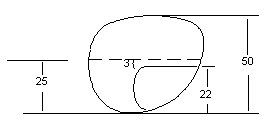< Page 6 of 17 > 1 2 3 4 5 6 7 8 9 10 11 12 13 14 15 16 17 Opthalmic Frames Minimum Blank Size & Vertical Decentration The formula for determining the smallest possible lens blank which will work for any given frame and PD combination is as follows: Minimum Blank Size (MBS) = (GCD – PD) + ED In the examples on the previous page, the ED is the same as the A measurement since the frame illustrated is round. Therefore, the theoretically smallest lens size that can be used in Example A is 44 mm. The theoretically smallest lens which can be used in Example B is equal to 48 mm. Example A: MBS = (64 – 64 ) + 44 = 44 Example B: MBS = (64 – 60) + 44 = 48 These minimum blank sizes are considered to be theoretical for two reasons: Two to three millimeters should always be added the the MBS to allow for imperfections such as chips or bubbles which may exist along the periphery of an uncut lens. Lens sizes are available from the manufacturers only in certain predetermined sizes, the diameters of which usually range from 60mm - 80 mm in 5-6 mm increments. It is necessary to calculate vertical decentration when determining where the line of a bifocal segment is positioned relative to the datum line of the frame. This may be expressed in the following formula. Vertical Decentration = seg height ­ (B measurment / 2)In the example to the left, since the B measurement = 50 mm the datum line is 25 mm from the bottom most portion of the lens. The seg height is 22 mm. Subtracting half the B measurement from the seg height results in : 22 – 25 = –3. Therefore the segment line is decentered 3 mm below the datum line, or would be commonly referred to as seg = 3 below. If the seg height were say, at 28 mm, the result would be + 3 mm positioning the seg line 3 mm above the datum line or 3 above. < Page 6 of 17 > 1 2 3 4 5 6 7 8 9 10 11 12 13 14 15 16 17